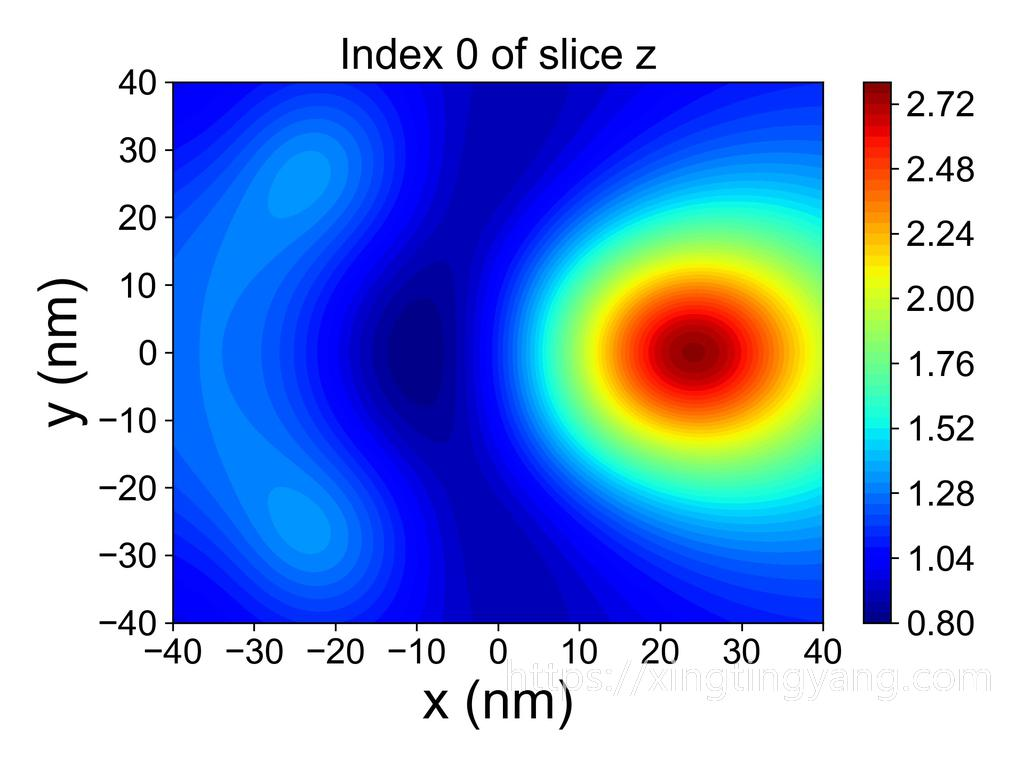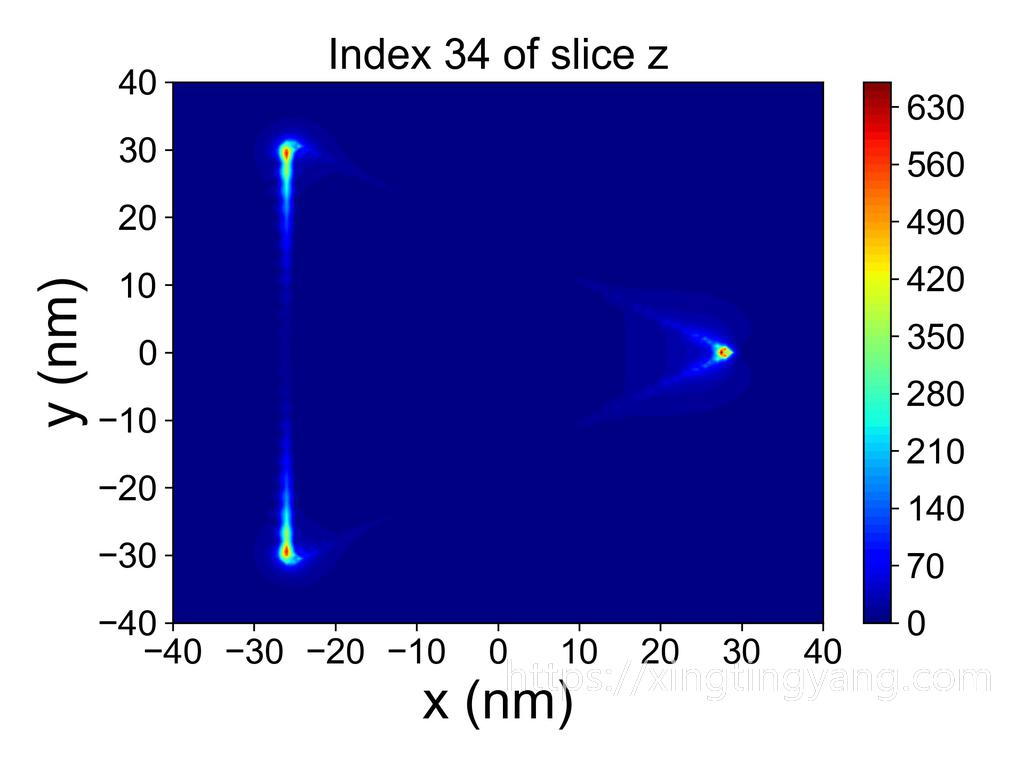# 2020/05/14 - Python处理FDTD Solutions导出数据

FDTD Solutions可以计算任意形状贵金属纳米颗粒的光学特性，其电场数据可以使用matlabsave方法导出，然后使用Python读取并画图。下面是一张3D的电场数据在Z方向上的某个切片。

## 三维数据

# f为h5py打开的文件对象
ME = f[keys[-1]]
E = np.array(ME['E']).transpose()


Ex = np.abs(E[:, 0, :]['real']+1j*E[:, 0, :]['imag'])
Ey = np.abs(E[:, 1, :]['real']+1j*E[:, 1, :]['imag'])
Ez = np.abs(E[:, 2, :]['real']+1j*E[:, 2, :]['imag'])

Ea = np.sqrt(np.power(Ex, 2)+np.power(Ey, 2)+np.power(Ez, 2))

index = np.argmax(Ea)
[i, j] = np.unravel_index(index, (sizes, sizes))
[m, n, k] = np.unravel_index(i, (sx, sy, sz))[::-1]


Ear = Ea[:, j].reshape((sx, sy, sz)).T

• 第一张切片• 最大的切片